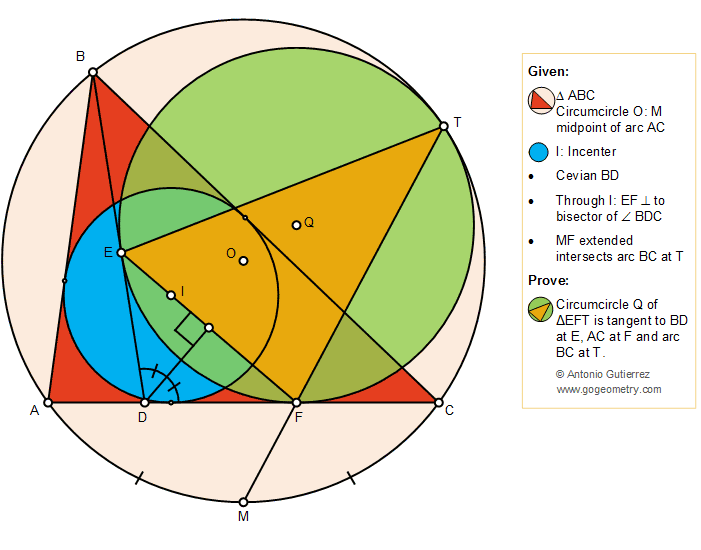Geometry Problem 1417: Triangle, Cevian, Circumcircle, Incenter, Perpendicular, Angle Bisector, Tangent Circles

Proposition

The figure below shows a triangle ABC with the incenter I, a cevian BD, the circumcircle O and M midpoint of arc AC. A line segment through I and perpendicular to the bisector of the angle BDC intersects BD at E, and AC at F. MF extended intersects arc BC at T. Prove that the circumcircle Q of the triangle EFT is tangent to BD at E, AC at F, and arc BC at T.Geometric Art of Problem 1417: Sketching, Typography, iPad Apps